﻿ 不同固结程度海洋软土动强度试验研究 Experimental Study on Dynamic Strength of Marine Soft Soil with Different Consolidation Degree

Hans Journal of Civil Engineering
Vol.06 No.02(2017), Article ID:19882,18 pages
10.12677/HJCE.2017.62013

Experimental Study on Dynamic Strength of Marine Soft Soil with Different Consolidation Degree

Shubin Li, Jinliang Song

School of Civil Engineering, Guangzhou University, Guangzhou Guangdong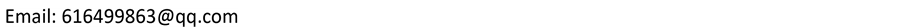Received: Feb. 17th, 2017; accepted: Mar. 11th, 2017; published: Mar. 14th, 2017ABSTRACT

In order to clarify the effects of cyclic stress ratio and frequency on dynamic strength of soft marine soil, a series of dynamic triaxial tests are performed. The test results show that: 1) the total strain decreases along with the increase of the frequency and over-consolidation ratio, but increases along with the increase of the cyclic stress ratio; 2) the softening index decreases along with the increase of vibration times and cyclic loading, but increases along with the increase of the frequency; 3) by conducting regression analysis on the softening curve and dynamic strength curve, we have obtained the softening coefficient on over-consolidation ratio and the empirical equation of dynamic strength.

Keywords:Marine Soft Soil, Over-Consolidation, Dynamic Strength, Dynamic Triaxial Test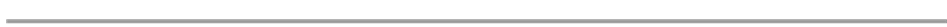1. 引言

2. 试验设备及方案

DDS-700微机控制电磁式振动三轴仪是完成本次试验的主要设备，是水利行业规范《土工试验规程》(SL237-2009)中振动三轴试验选定的设备，可用于测定各种土的动力特性指标。

3. 动应变-振次曲线分析

3.1. 循环应力比的影响Table 1. Physical-mechanical properties of soft soil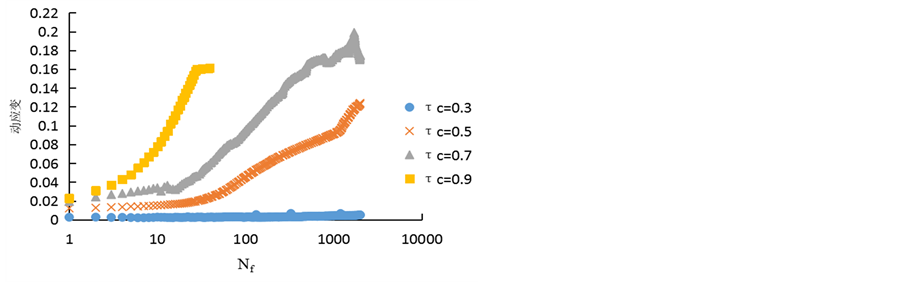(a) OCR = 1, f = 1 Hz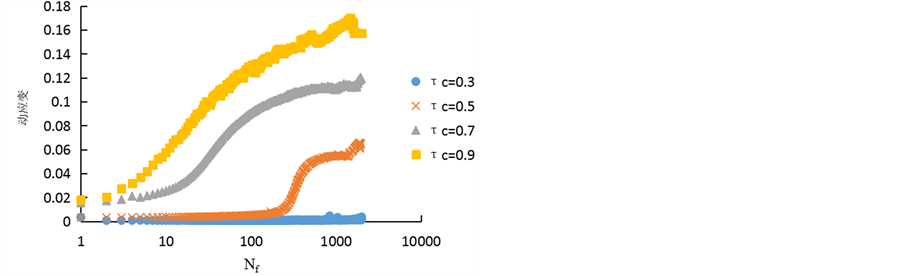(b) OCR = 2, f = 1 Hz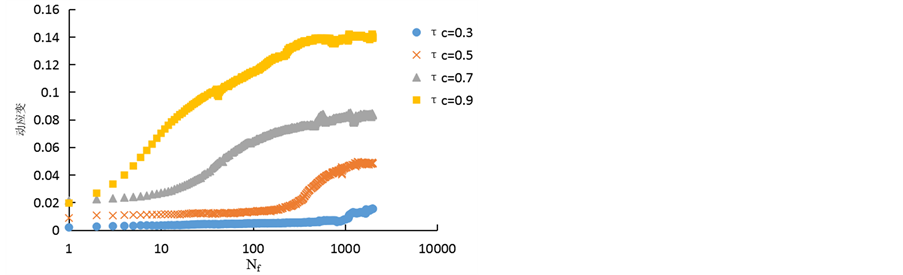(c) OCR = 4, f = 1 Hz

Figure 1. Relationship curves between dynamic strain and cyclic number at different cyclic stress ratio

3.2. 频率的影响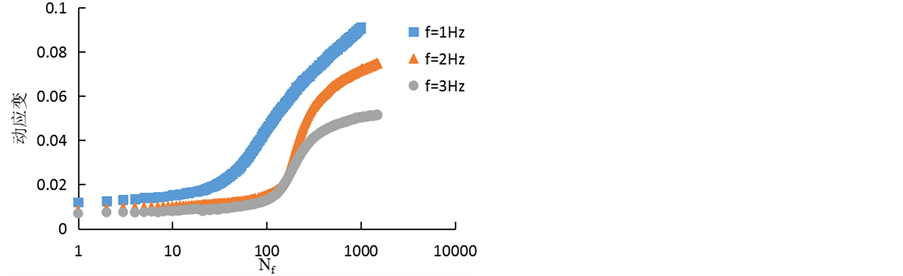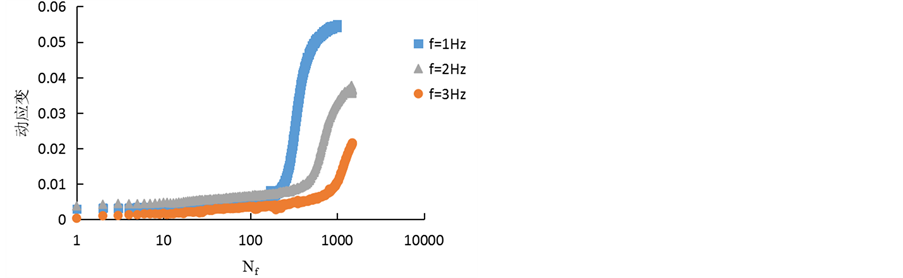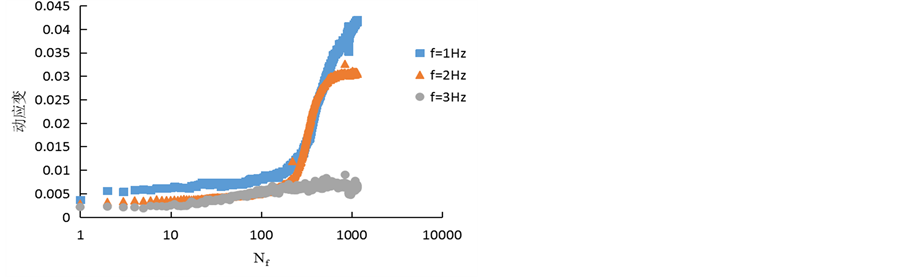(a) OCR = 1,(b) OCR = 2,(c) OCR = 4,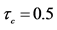Figure 2.Relationship curves between dynamic strain and cyclic number at different frequency

3.3. 超固结比的影响

4. 最大动应变分析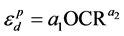(1)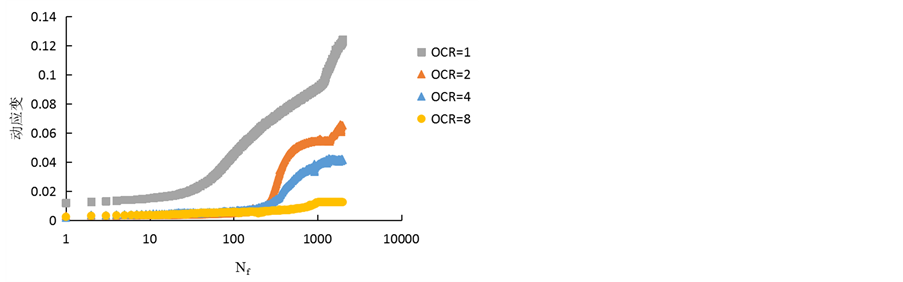Figure 3. Relationship curves between dynamic strain and cyclic number at different over consolidation ratio (f = 1 Hz,)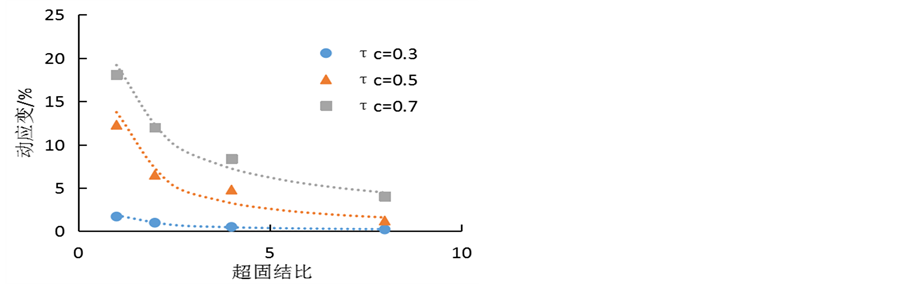Figure 4. Relationship curves between dynamic axial strain (the 1500 th) and over-consolidation ratio at different cyclic stress ratio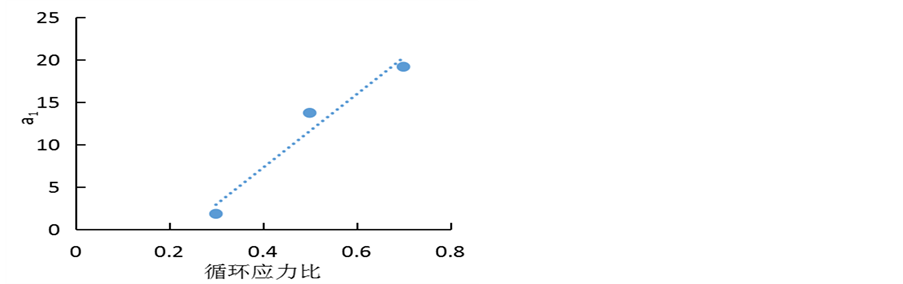Figure 5. Relationship curves of a1 vs. cyclic stress ratioFigure 6. Relationship curves of a2 vs. cyclic stress ratio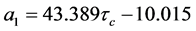(2)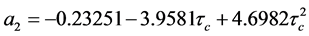(3)(4)

5. 振次-动应变模型建立与参数分析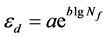(5)

(1) 关于频率的回归公式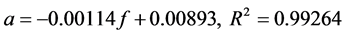(6) (7)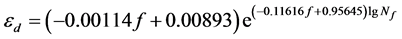(8)

(2)关于超固结比的回归公式(9)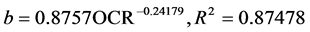(10)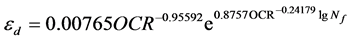(11)

(3)关于循环应力比的回归公式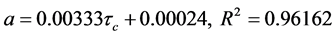(12)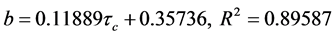(13)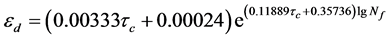(14)

6. 软化特性分析(15)

6.1. 循环应力比的影响

6.2. 频率的影响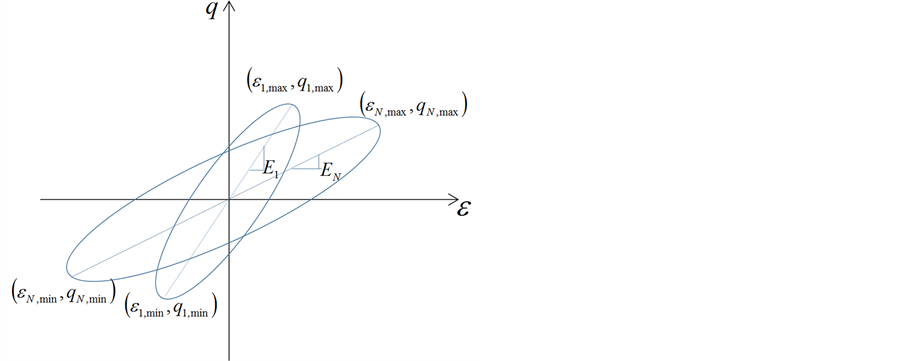Figure 7. Relationship curves between stresses and strains (the first and nth) under cyclic loading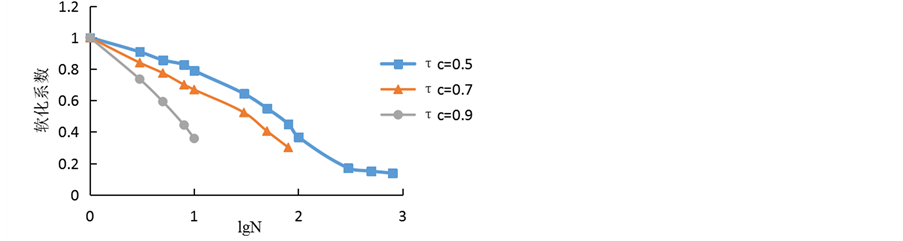(a) OCR = 1, f = 1 Hz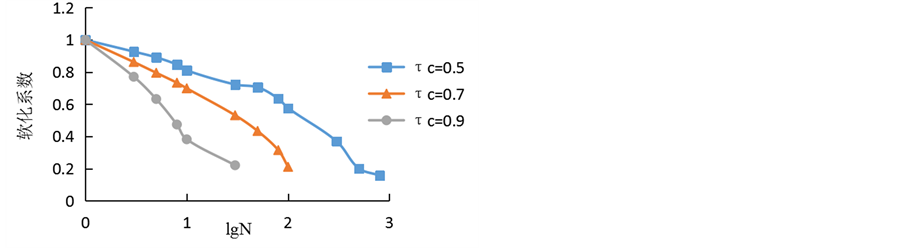(b) OCR = 2, f = 1 Hz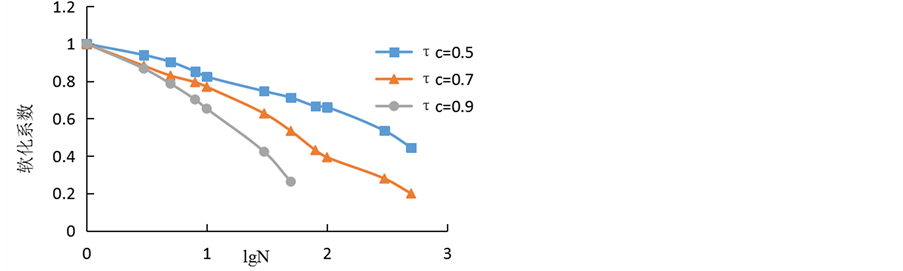(c) OCR = 4, f = 1 Hz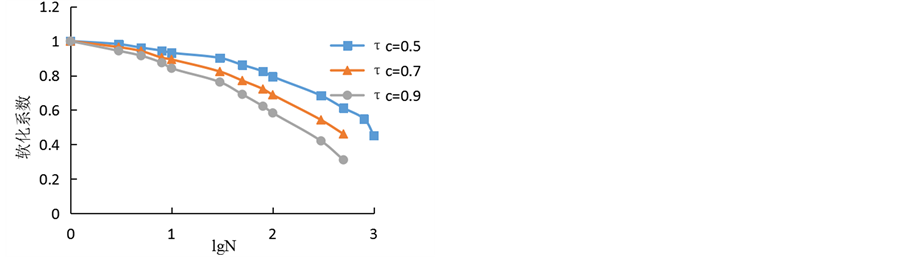(d) OCR = 8, f = 1 Hz

Figure 8. Relationship curves between softening index and cyclic number at different cyclic stress ratio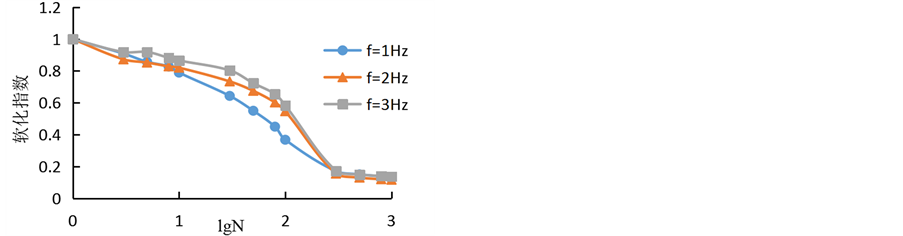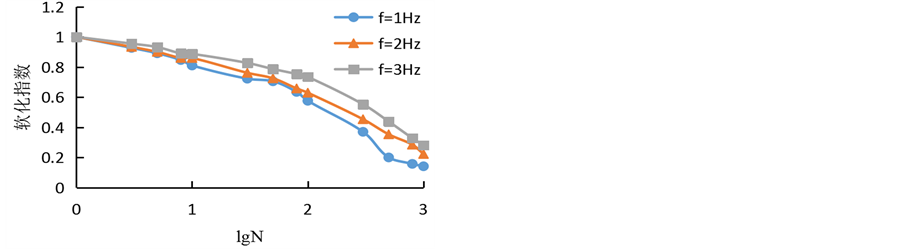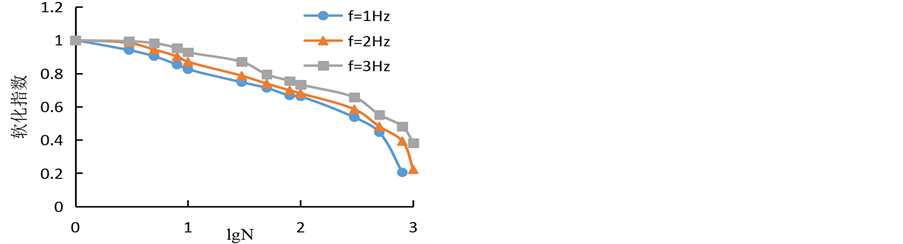(a) OCR = 1,(b) OCR = 2,(c) OCR = 4,Figure 9.Relationship curves between softening index and cyclic number at different frequency

6.3. 超固结比的影响

7. 软化模型建立及参数分析(16)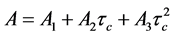(17)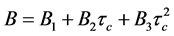(18)f = 1 Hz，Figure 10.Relationship curves between softening index and cyclic number at over consolidation ratio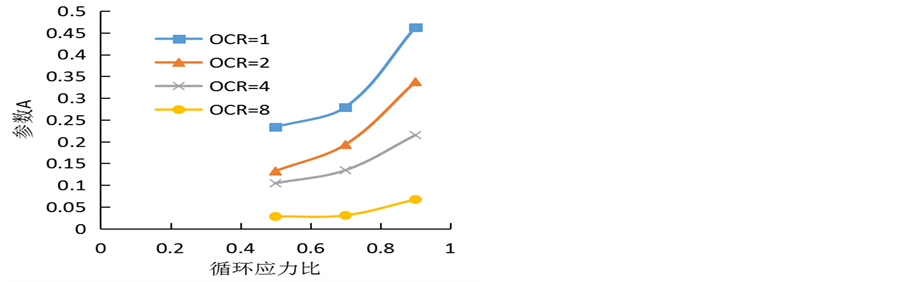Figure 11. Relationship curves of A vs. cyclic stress ratioFigure 12. Relationship curves of B vs. cyclic stress ratio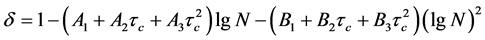(19)Table 6. Parameter A1, A2, A3, B1, B2 and B3 fitting results

8. 动强度分析

8.1. 破坏准则分析

8.2. 超固结比的影响

8.3. 频率的影响

9. 动强度模型的建立及参数分析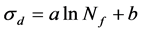(20)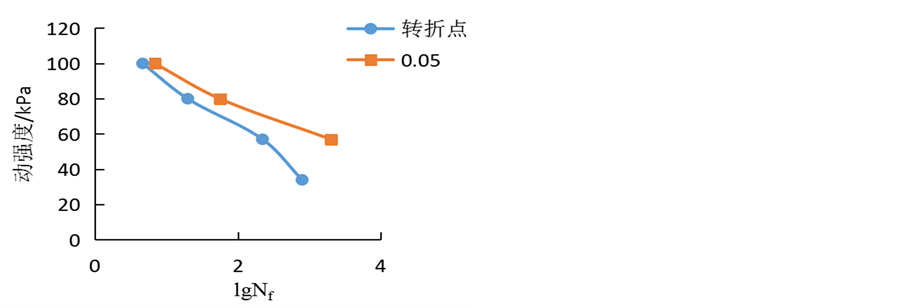(a) OCR = 1, f = 1 Hz (b) OCR = 2, f = 1 Hz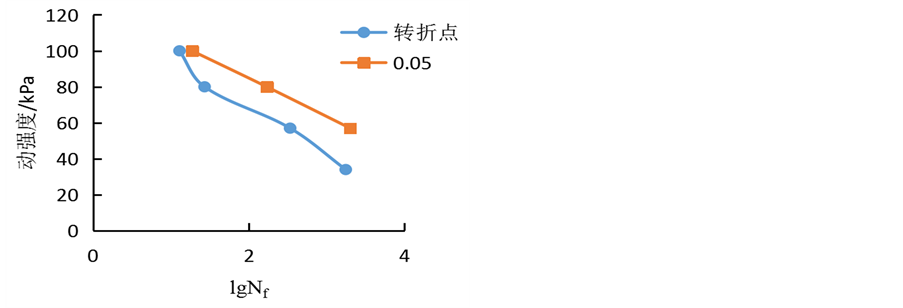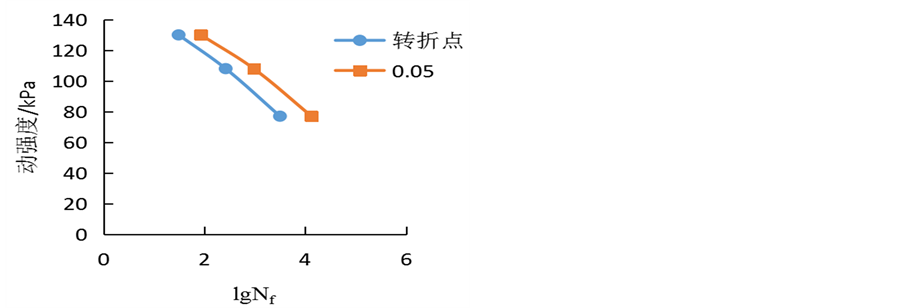(c) OCR = 4, f = 1 Hz (d) OCR = 1, f = 2 Hz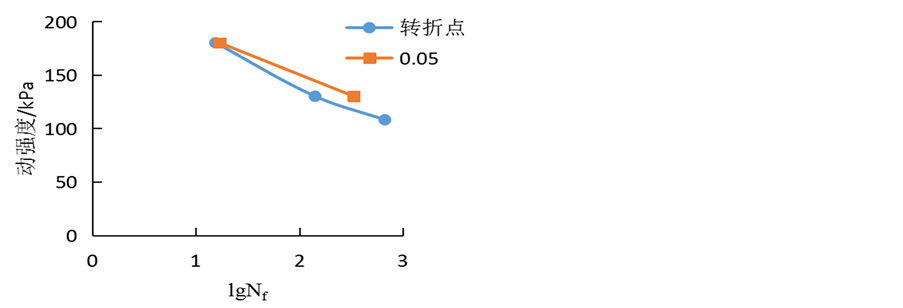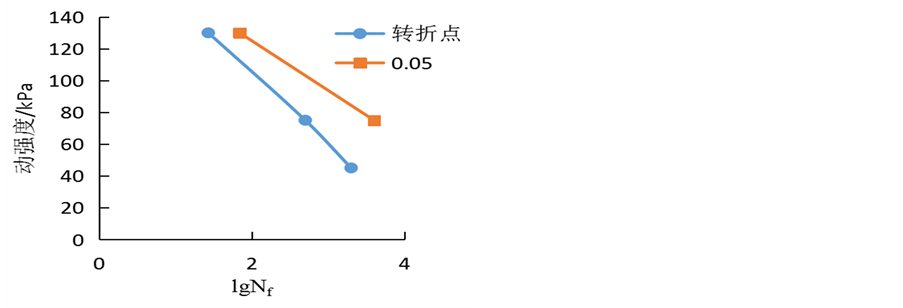(e) OCR = 2, f = 2 Hz (f) OCR = 4, f = 2 Hz

Figure 13. Dynamic strength curve under two different strain failure criteria(21)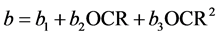(22)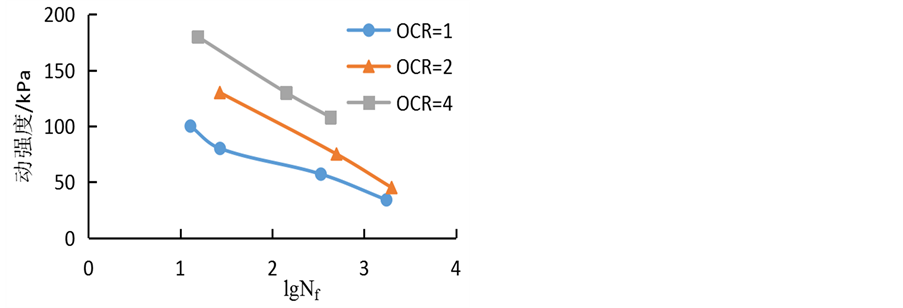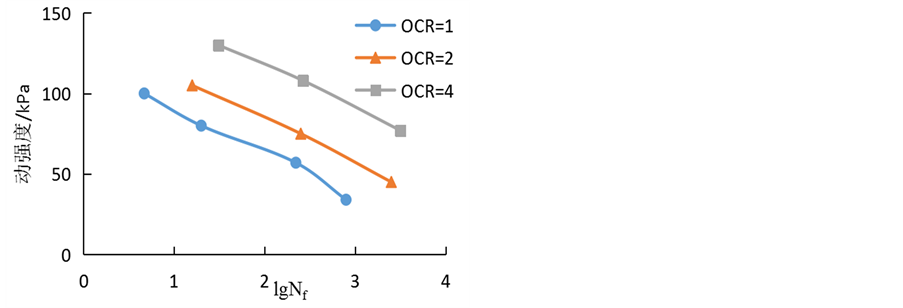(a) f = 1 Hz(b) f = 2 Hz

Figure 14. Dynamic strength curve at different over consolidation ratio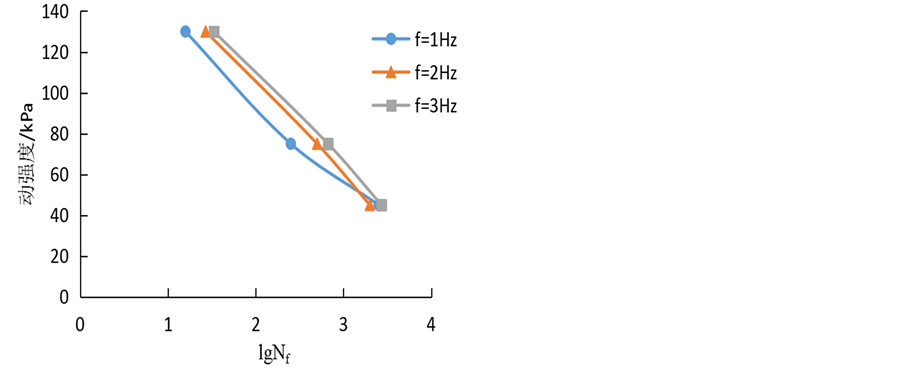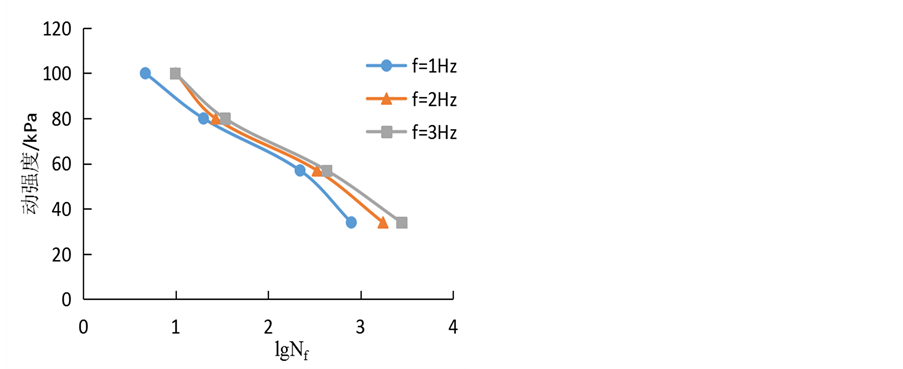(a) OCR = 1(b) OCR = 2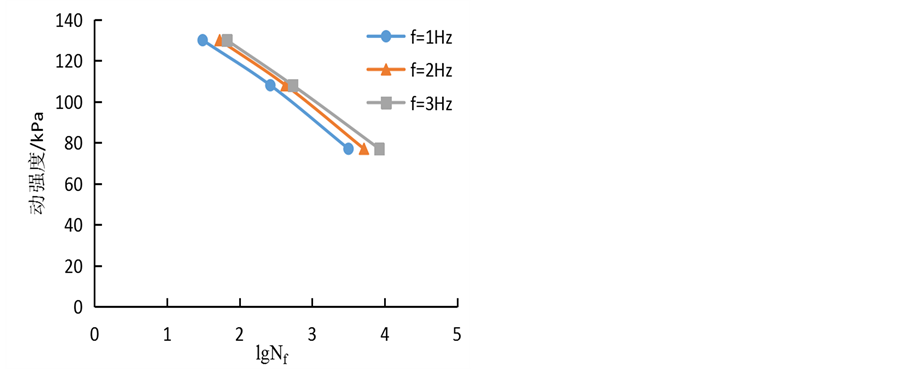(c) OCR = 4

Figure 15. Dynamic strength curve at different frequencyFigure 16. Relationship curves of a vs. over consolidation ratio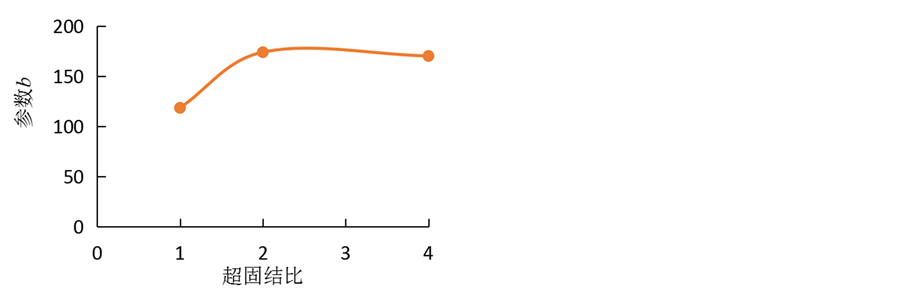Figure 17. Relationship curves of b vs. over consolidation ratio(23)

10. 结论

(1) 在循环荷载作用下，随着振动次数的增加，土体动应变不断增大，同时变化曲线存在两个应变转折点，加载初期，动应变缓慢增大，达到第一个转折点之后，总应变迅速增大，达到第二个转折点，动应变增长速度减缓，最后趋于稳定。第二个转折点前的曲线可以用指数函数进行拟合。

(2) 在同一振次下，循环应力比越大、动应变越大；超固结比越大、动应变越小；频率越大、动应变也越小。在相同的频率下，试样在不同循环应力比下产生的最大动应变随超固结比的增大而降低，且下降规律相同，可以采用幂函数进行拟合。

(3) 分析软化特性，发现软化指数随着振次的增加而降低，循环应力比越大，软化越快，超固结比越大，软化越慢，但频率对软化指数影响不大。建立软化模型并分析其参数，即可得到其关于振次的公式。

(4) 以第一个应变转折点和总应变5%为破坏标准进行分析，发现用转折点作为破坏标准更安全。以转折点应变作为破坏强度标准，通过绘出不同超固结比和频率的动强度曲线，可以发现，破坏振次越大，动强度越低，超固结比越大，动强度越大，频率越大，动强度也越大，但不明显。建立动强度模型并分析其参数，即可以得到关于超固结比的动强度经验公式。

Experimental Study on Dynamic Strength of Marine Soft Soil with Different Consolidation Degree[J]. 土木工程, 2017, 06(02): 110-127. http://dx.doi.org/10.12677/HJCE.2017.62013

1. 1. 聂影, 栾茂田, 唐小微, 等. 超固结黏土单调和耦合循环的剪切特性研究[J]. 岩土力学, 2009, 30(9): 2616-2622.

2. 2. 孙磊, 王军, 孙宏磊, 等. 循环围压对超固结黏土变形特性影响试验研究[J]. 岩石力学与工程学报, 2015(3): 594- 600.

3. 3. Mayne, P.W. (1988) Determining OCR in Clays from Laboratory Strength. Journal of Geotechniccal, 114, 22-31.

4. 4. Azzouz, A.S., Malek, A.M. and Baligh, M.M. (1989) Cyclic Behavior of Clays in Undrained Simple Shear. American Society of Civil Engineers, 115, 637-657. https://doi.org/10.1061/(asce)0733-9410(1989)115:5(637)

5. 5. Imai, G. and Xie, C. (1991) Endochronic Modeling for Cyclic Behavior of Overconsolidated Clays. Soils & Foundations, 31, 103-114. https://doi.org/10.3208/sandf1972.31.4_103

6. 6. Jiang, M.M., Cai, Z.Y., Cao, P., et al. (2010) Effect of Cyclic Loading Frequency on Dynamic Properties of Marine Clay. Soil Dynamics and Earthquake Engineering (GSP 201). Proceedings of the 2010 Geo Shanghai International Conference, 340-346. https://doi.org/10.1061/41102(375)29

7. 7. Larew, H.G. and Leonards, G.A. (1962) A Repeated Load Strength Criterion. Highway Research Board Proceedings.

8. 8. Idriss, I.M., Dobry, R. and Singh, R.D. (1978) Nonlinear Behavior of Soft Clays during Cyclic Loading. Journal of the Geotechnical Engineering Division, 104, 1427-1447.

9. 9. Yasuhara, Y., Hyde, A.F.L., Toyata, N., et al. (1998) Cyclic Stiffness of Plastic Silt with an Initial Dranied Shear Stress. Proceedings of Geotechnique Symposium in Print (SIP) on Prefailure Deformation Behavior of Geomterials, London, 371-382.

10. 10. 王军, 蔡袁强, 徐长节, 等. 循环荷载作用下饱和软黏土应变软化模型研究[J]. 岩石力学与工程学报, 2007, 26(8): 1713-1719.